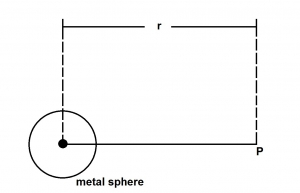The diagram above illustrates an isolated metal sphere carrying charge W in a medium whose permittivity is so. The magnitude of the electric field intensity at P can be expressed as

A. Q^2/(4πεor^2)
B. Q/(4πεor^2)
C. Q/(4πεor)
D. Q^2/(4πεor^2)
E. Q/(4πεor)^2